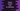# Python program to convert inch to centimeter## Python program to convert inch to centimeter:

In this post, we will learn how to convert a value in inch to centimeter in python. This program will take one value as input from the user in inches, convert it to centimeter and print it out. If you know the conversion formula, you can do any conversion programmatically.

I will show you how to convert one inch value to centimeter and also I will show you how to format the output to show upto a position after the decimal.

## How to convert Inch to centimetre:

This program will take the inch value as input from the user. 1 inch is equal to 2.54 centimeter. So, if we multiply the user input value with 2.54, we will get the centimeter.

## Python program to convert inch to centimetre:

Let’s write down the python program now. Below is the complete program:

``````inch_value = int(input('Enter the value in inch: '))
cm_value = 2.54 * inch_value

print('{}″ = {}cm'.format(inch_value, cm_value))``````

### Explanation:

In this program,

• We are taking the value of inch as input from the user. We are reading this value as int and storing it in inch_value.
• This value is multiplies by 2.54 to calculate the centimeter value and it is stored in cm_value.
• The last line is printing these values to the user.

### Output:

If you run this program, it will print output as like below:

``````Enter the value in inch: 30
10″ = 25.4cm

Enter the value in inch: 30
20″ = 50.8cm

Enter the value in inch: 30
30″ = 76.2cm``````

## Method 2: By using a different function:

We can also write a different function to simplify this. Writing a different function is always helpful because we can call it from different parts of a program. Let me show you how to do that:

``````def inch_to_cm(inch):
return inch * 2.54

inch_value = int(input('Enter the value in inch: '))

print('{}″ = {}cm'.format(inch_value, inch_to_cm(inch_value)))``````

This is doing the same process. The only difference is that we created inchtocm to calculate the centimeter value for a given inch value. This method takes the inch value as its argument, calculates the centimeter value by multiplying it with 2.54 and returns that.

In the last print statement, we are calling inchtocm to calculate the cm value of a given inch value.

This program will give similar output as the above one.